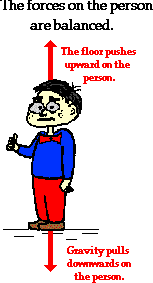http://farm8.staticflickr.com/7003/6440972687_275d53950e_z.jpg
The Forces are with you

Trampolines are a wonderful way to exhibit  Newton’s Laws of motion.I. Every object in a state of uniform motion tends to remain in that state of motion unless an external force is applied to it.
In this case the trampoline is the external force and the jumper is the external force on the trampoline.
II. The relationship between an object's mass m, its acceleration a, and the applied force F is F = ma. Acceleration and force are vectors (as indicated by their symbols being displayed in slant bold font); in this law the direction of the force vector is the same as the direction of the acceleration vector.
If the boy was sitting on the trampoline with his buddies then forces would be balanced and they would all be at equilibrium as long as none of them moved at all.

III. For every action there is an equal and opposite reaction.

Jump on a trampoline and you will see that this is true.

 Home Potential Energy Forces Equilibrium KineticEnergy Bibliography